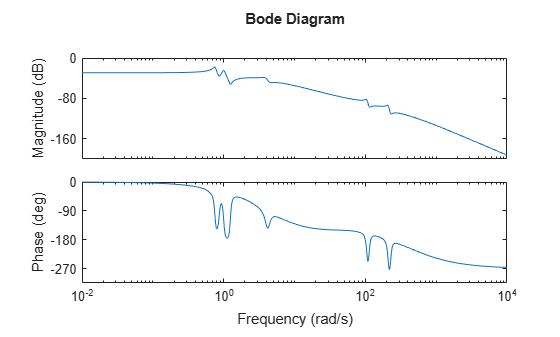# hsvd

(Not recommended) Hankel singular values of dynamic system

`hsvd` is not recommended. Use `balred` instead. For more information, see Compatibility Considerations.

## Syntax

```hsv = hsvd(sys) hsv = hsvd(sys,opts) [hsv,baldata] = hsvd(___) hsvd(___) ```

## Description

`hsv = hsvd(sys)` computes the Hankel singular values `hsv` of the dynamic system `sys`. In state coordinates that equalize the input-to-state and state-to-output energy transfers, the Hankel singular values measure the contribution of each state to the input/output behavior. Hankel singular values are to model order what singular values are to matrix rank. In particular, small Hankel singular values signal states that can be discarded to simplify the model (see `balred`).

For models with unstable poles, `hsvd` only computes the Hankel singular values of the stable part and entries of `hsv` corresponding to unstable modes are set to `Inf`.

`hsv = hsvd(sys,opts)` computes the Hankel singular values using options that you specify using `hsvdOptions`. Options include offset and tolerance options for computing the stable-unstable decompositions. The options also allow you to limit the HSV computation to energy contributions within particular time and frequency intervals. See `balredOptions` for details.

`[hsv,baldata] = hsvd(___)` returns additional data to speed up model order reduction. You can use this syntax with any of the previous combinations of input arguments.

`hsvd(___)` displays a Hankel singular values plot.

## Examples

collapse all

Create a system with a stable pole very near to 0, and display the Hankel singular values.

```sys = zpk([1 2],[-1 -2 -3 -10 -1e-7],1); hsv = hsvd(sys)```
```hsv = 5×1 105 × 1.6667 0.0000 0.0000 0.0000 0.0000 ```

Notice the dominant Hankel singular value with magnitude $1{0}^{5}$, which is so much larger that the significant digits of the other modes are not displayed. This value is due to the near-unstable mode at $s=1{0}^{-7}$. Use the `'Offset'` option to treat this mode as unstable.

```opts = hsvdOptions('Offset',1e-7); hsvu = hsvd(sys,opts)```
```hsvu = 5×1 Inf 0.0688 0.0138 0.0024 0.0001 ```

The Hankel singular value of modes that are unstable, or treated as unstable, is returned as `Inf`. Create a Hankel singular-value plot while treating this mode as unstable.

`hsvd(sys,opts)`
```ans = 5×1 Inf 0.0688 0.0138 0.0024 0.0001 ```

The unstable mode is shown in red on the plot.

By default, `hsvd` uses a linear scale. To switch the plot to a log scale, right-click on the plot and select Y Scale > Log. For information about programmatically changing properties of HSV plots, see `hsvplot`.

Compute the Hankel singular values of a model with low-frequency and high-frequency dynamics. Focus the calculation on the high-frequency modes.

Load the model and examine its frequency response.

```load modeselect Gms bodeplot(Gms)````Gms` has two sets of resonances, one at relatively low frequency and the other at relatively high frequency. Compute the Hankel singular values of the high-frequency modes, excluding the energy contributions to the low-frequency dynamics. To do so, use `hsvdOptions` to specify a frequency interval above 30 rad/s.

```opts = hsvdOptions('FreqInterval',[30 Inf]); hsvd(Gms,opts)```
```ans = 18×1 10-4 × 0.6237 0.4558 0.3183 0.2468 0.0895 0.0847 0.0243 0.0028 0.0000 0.0000 ⋮ ```

## Tips

To create a Hankel singular-value plot with more flexibility to programmatically customize the plot, use `hsvplot`.

## Version History

Introduced before R2006a

expand all

Not recommended starting in R2021a